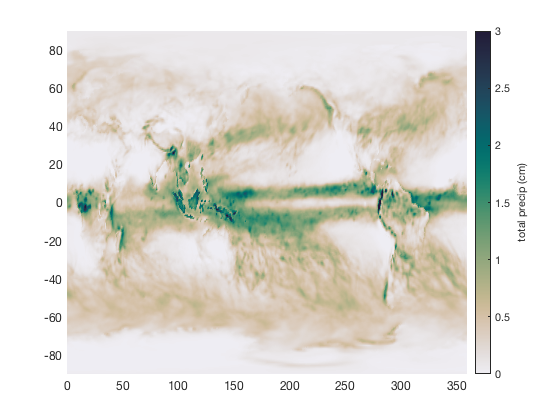# monthly documentation

The monthly function calculates statistics of a variable for specified months of the year.

See also: season, climatology, sinefit, sineval, and sinefit_bootstrap.

## Syntax

```Xm = monthly(X,t,months)
Xm = monthly(...,'dim',dimension)
Xm = monthly(...,@fn)
Xm = monthly(...,'omitnan')
Xm = monthly(...,options)```

## Description

Xm = monthly(X,t,months) returns the mean of X for all months specified by the numbers 1 through 12. For example, if months is specified as 1, Xm will be the mean of all X values for January. If months is [12 1 2], Xm will be the mean of all DJF. Times t correspond to X and can be in datenum, datetime, or datestr format.

Xm = monthly(...,'dim',dimension) specifies a dimension of operation. By default, if X is a 1D array, the t is assumed to correspond to the first nonsingleton dimension of X; if X is a 2D matrix, the t is assumed to correspond to the rows of X; and if X is a 3D matrix, time is assumed to be dimenion 3 of X.

Xm = monthly(...,@fn) specifies any function such as @max, @std, or your own anonymous function to apply to X. The default function is @mean.

Xm = monthly(...,'omitnan') ignores NaN values in the calculation.

Xm = monthly(...,options) specifies any optional inputs, which will depend on the @fn applied to the data.

## Example 1: Spring sea ice extent

What's the average springtime Antarctic sea ice extent? Let's load the example time series and plot it to get a sense of the question we're asking:

```% Load the sample data:

% Plot sea ice extent as a function of day of year:
plot(doy(t),extent_S,'.')

hold on    % allows adding to the plot
box off    % removes frame
axis tight % removes extra space
xlabel 'day of year'
ylabel 'sea ice extent (10^6 km^2)'
ntitle 'Antarctic sea ice'

% Highlight the SON months:
vfill(doy('sept 1'),doy('nov 30'),'b','facealpha',0.1)
```Above, the daily sea ice extent from 1978 to present is plotted as a function of day of year. The doy function converts dates to day of year, ntitle creates the title that's tucked nicely inside the axes, and hfill creates the shaded region from Sept 1 to Nov 30.

In the plot above, we've implicitly defined spring as September through November (SON) by shading that region. Now we can get the mean sea ice extent for those months using the monthly function, specifying months 9, 10, and 11 as our months of interest:

```monthly(extent_S,t,[9 10 11])
```
```ans =
17.53
```

The mean spring Antarctic sea ice extent is about 17.5 million square kilometers. We can plot that if you'd like:

```plot([doy('sept 1') doy('nov 30')],[17.5 17.5],'r')

text(doy('sept 1'),17.5,' SON mean','color','r','vert','top')
```It's worth noting here that there's another way we could have arrived at a similar answer. We could have used climatology to get the daily climatology for the sea ice time series, like this:

```[extent_S_clim,t_clim] = climatology(extent_S,t);

% Plot the daily climatology as a black line:
plot(t_clim,extent_S_clim,'k','linewidth',2)
```Then the mean SON value can be obtained as the mean of all daily climatology values from doy('sept 1') to doy('nov 30'):

```mean(extent_S_clim(doy('sept 1'):doy('nov 30')))
```
```ans =
17.56
```

## Example 2: Precipitation totals:

For this example, load some ERA-Interim reanalysis data that contains monthly precipitation totals for 2017:

```filename = 'ERA_Interim_2017.nc';
```

What was the total amount of precipitation that fell in March through May of 2017?

```MAM_sum = monthly(tp,t,3:5,@sum);

figure
pcolor(lon,lat,MAM_sum'*100)
cmocean rain
cb = colorbar;
ylabel(cb,'total precip (cm)')
caxis([0 3])
```Since the 2017 dataset contains only 1 year of data, taking the March-May sum is exactly the same as if we had added those monthly grids together manually:

```% Compare monthly solution to manually adding things up:
isequal(MAM_sum, tp(:,:,3)+tp(:,:,4)+tp(:,:,5))
```
```ans =
logical
1
```

## Example 3: Interannual variability

How predictable are Marches in the Pacific? By that I mean, will the sea surface temperature in March of next year be about the same as it was in March of last year, or is there a tremendous amount of variability between Marches?

To answer this question, apply the monthly function to the sample pacific_sst dataset, which contains 67 years of data:

```load pacific_sst

mar_var = monthly(sst,t,3,@var);

figure
imagescn(lon,lat,mar_var)
caxis([0.05 2])
cmocean amp
```What we see in the plot above is that along coasts, there is some variance in March sea surface temperature as it can change significantly from year to year, but in the southeastern Pacific, March SST variance is quite low, meaning Marches are more predictable there from climatology.

Let's put the mean March SST together on a map with the variance of March SST:

```% Calculate mean SST for all Marches:
mar_mean = monthly(sst,t,3);

figure
imagescn(lon,lat,monthly(sst,t,3))
cmocean thermal
cb = colorbar;
ylabel(cb,'mean March SST (\circC)')

% plot variance as contours:
hold on
contour(lon,lat,mar_var,0.5:0.1:8,'k')
```## Author Info

This function is part of the Climate Data Toolbox for Matlab. The function and supporting documentation were written by Chad A. Greene of the University of Texas at Austin.